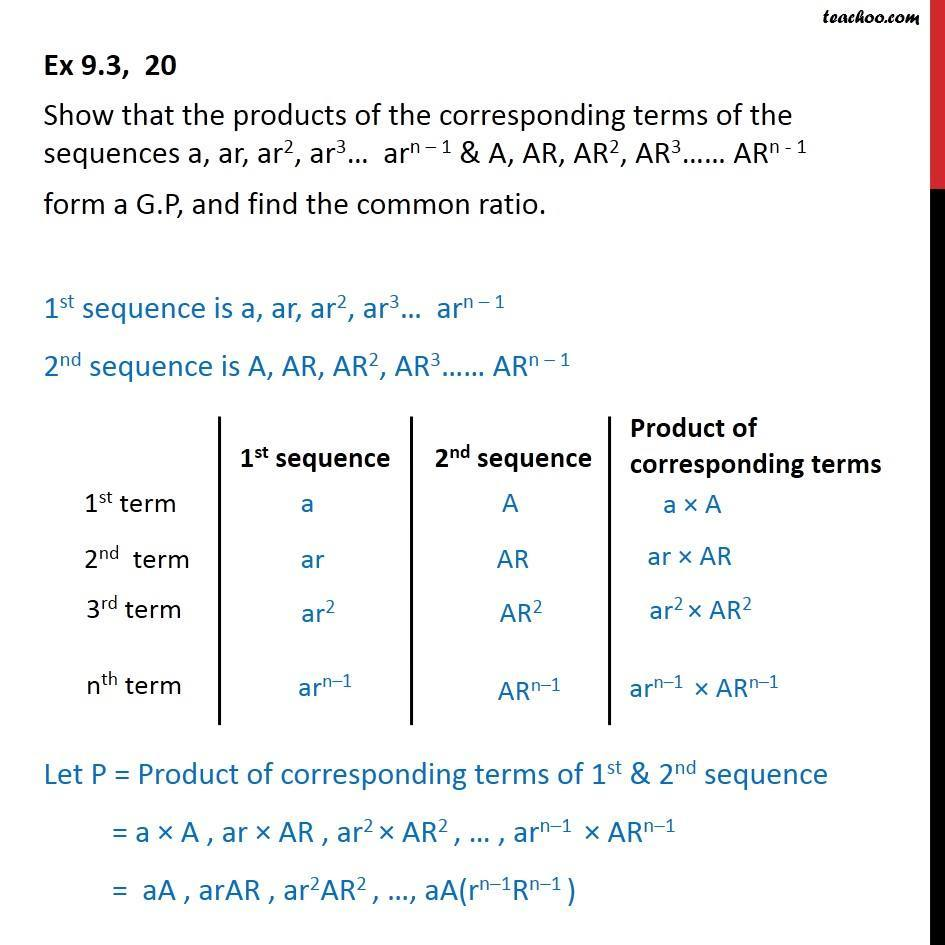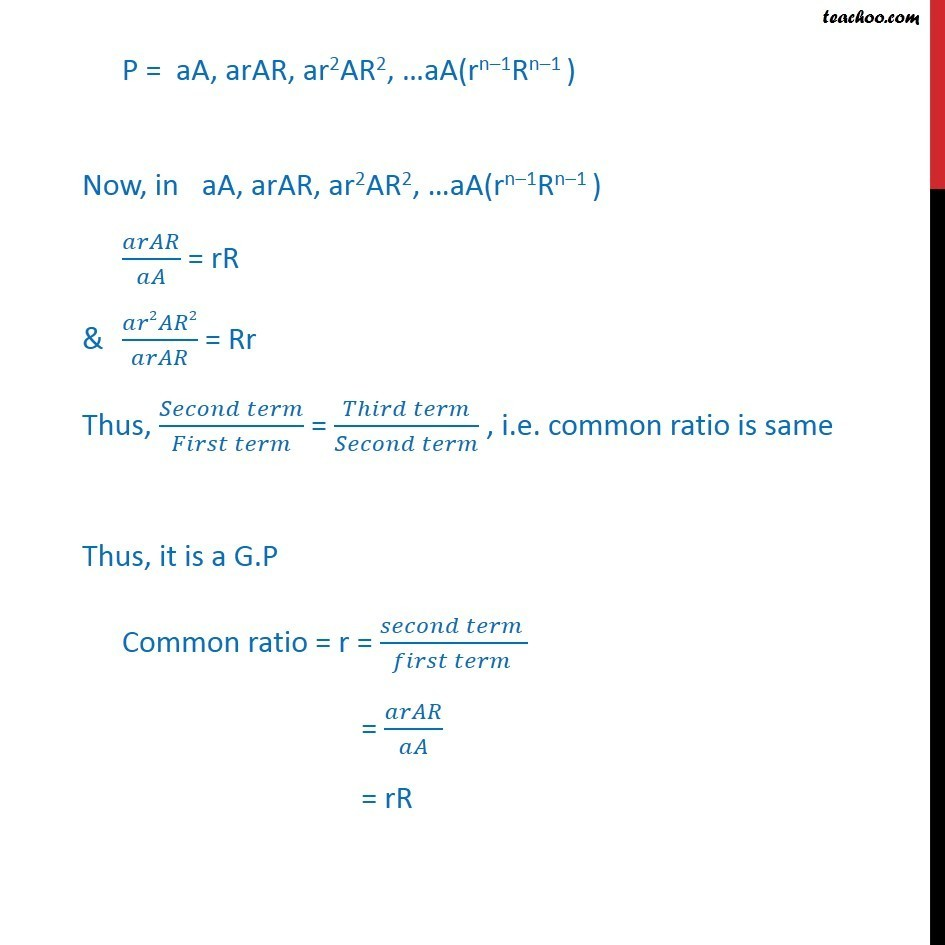1. Chapter 9 Class 11 Sequences and Series
2. Serial order wise
3. Ex 9.3

Transcript

Ex 9.3, 20 Show that the products of the corresponding terms of the sequences a, ar, ar2, ar3… arn – 1 & A, AR, AR2, AR3…… ARn - 1 form a G.P, and find the common ratio. 1st sequence is a, ar, ar2, ar3… arn – 1 2nd sequence is A, AR, AR2, AR3…… ARn – 1 Let P = Product of corresponding terms of 1st & 2nd sequence = a × A , ar × AR , ar2 × AR2 , … , arn–1 × ARn–1 = aA , arAR , ar2AR2 , …, aA(rn–1Rn–1 ) P = aA, arAR, ar2AR2, …aA(rn–1Rn–1 ) Now, in aA, arAR, ar2AR2, …aA(rn–1Rn–1 ) 𝑎𝑟𝐴𝑅/𝑎𝐴 = rR & 𝑎𝑟2𝐴𝑅2/𝑎𝑟𝐴𝑅 = Rr Thus, (𝑆𝑒𝑐𝑜𝑛𝑑 𝑡𝑒𝑟𝑚)/(𝐹𝑖𝑟𝑠𝑡 𝑡𝑒𝑟𝑚) = (𝑇ℎ𝑖𝑟𝑑 𝑡𝑒𝑟𝑚)/(𝑆𝑒𝑐𝑜𝑛𝑑 𝑡𝑒𝑟𝑚) , i.e. common ratio is same Thus, it is a G.P Common ratio = r = (𝑠𝑒𝑐𝑜𝑛𝑑 𝑡𝑒𝑟𝑚 )/(𝑓𝑖𝑟𝑠𝑡 𝑡𝑒𝑟𝑚 ) = 𝑎𝑟𝐴𝑅/𝑎𝐴 = rR

Ex 9.3